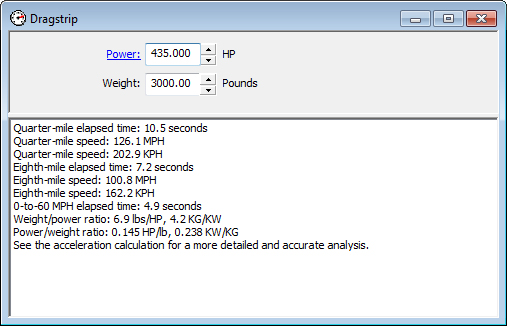## Dragstrip Calculation

The dragstrip calculation provides a quick and easy, but surprisingly accurate, calculation of quarter-and eighth-mile acceleration time and trap speed, and zero-to-sixty MPH acceleration time. The calculation is based solely on power-to-weight ratio, which is also displayed. Proper set-up such as gearing and traction are assumed to save you from having to enter a lot of information in order to get an acceleration estimate.

For a more detailed acceleration calculation, see acceleration input, acceleration table, and acceleration plot.Enter vehicle power and weight. Calculate quarter-mile elapsed time, quarter-mile trap speed, eighth-mile elapsed time, eighth-mile trap speed, 0-to-60 MPH elapsed time, weight to power ratio, and power to weight ratio.